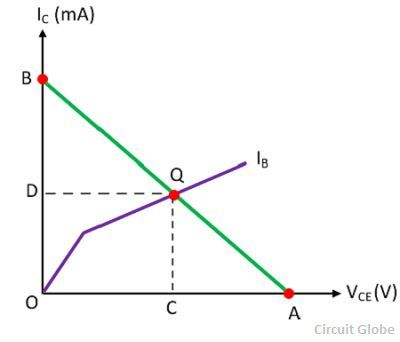# Operating Point in Transistor

Definition: The point which is obtained from the values of the I(collector current) or VCE (collector-emitter voltage) when no signal is given to the input is known as the operating point or Q-point in a transistor. It is called operating point because variations of IC (collector current) and VCE (collector-emitter voltage) takes place around this point when no signal is applied to the input.

The operating point is also called quiescent (silent) point or simply Q-point because it is a point on IC – VCE characteristic when the transistor is silent or no input signal is applied to the circuit. The operating point can be easily obtained by the DC load line method. The DC load line is explained belowLet, determines the operating point of particular base circuit current IB. According to the load line condition, the OA = VCE = VCC and OB = IC = VCC/RC is shown on the output characteristic curve above. The point Q is the operating point where the DC load line intersects the base current IB at the output characteristic curves in the absence of input signal.

Where IC= OD mA

VCE = OC volts.

The position of the Q-point depends on the applications of the transistor. If the transistor is used as a switch then for open switch the Q-point is in the cutoff region, and for the close switch, the Q-point is in the saturation region. The Q-point lies in the middle of the line for the transistor which operates as an amplifier.

Note: In saturation region, both the collector base region and the emitter-base region are in forward biased and heavy current flow through the junction. And the region in which both the junctions of the transistor are in reversed biased is called the cut-off region.